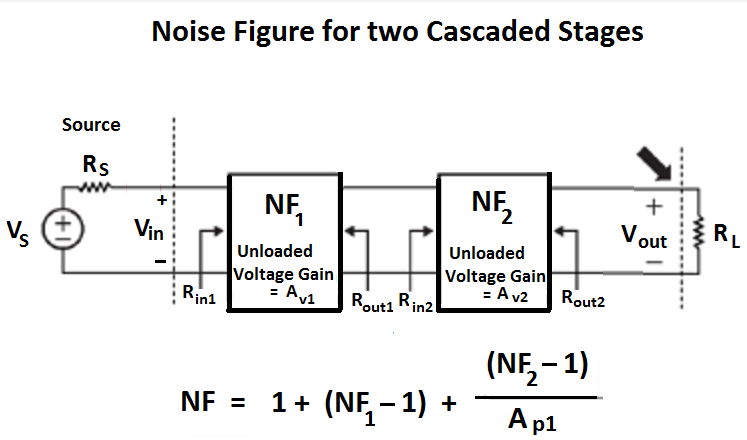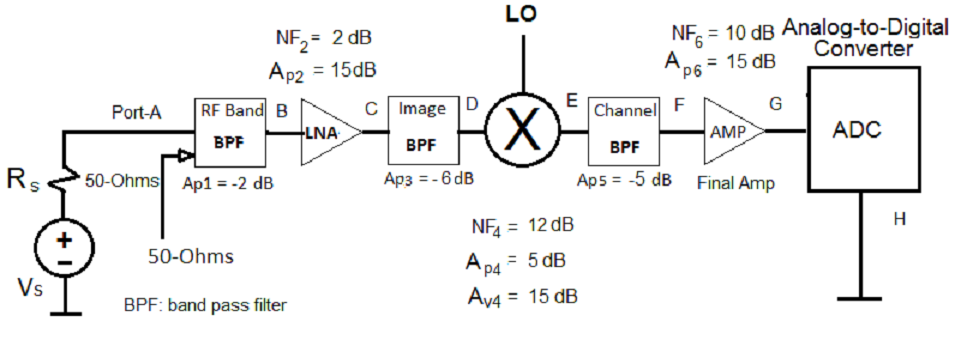## Combined Blocks of Signal Chain Path

Marcel Merchat
RF and Microwave Engineer

### The noise figure of a receiver is determined by the noise in the chain of circuit blocks in the signal path. Typical blocks in the chain are as follows:

• RF filter
• Low noise amplifier (LNA)
• Mixer
• Intermediate Frequency Filter (IF)
• Analog-to-Digital Converter (quantization noise)
• Coding Gain (This reduces the noise.)

## Combined Noise Figure of Two Circuit BlocksVs: input signal voltage Rs: output impedance of signal source Vin1: input voltage for first block Rin1: input impedance of first block NF1: Noise figure of first block Avs1: Voltage gain of first block Ap1: Power gain of first block Rout1:output impedance of first block Rin2: input impedance of second block NF2 : Noise figure of second block Avs2: Voltage gain of second block Ap2 : Power gain of second block Rout2: Output impedance of second block Vs2 : Output voltage for second block

## RF Filter Block

#### We calculate the noise figure of a filter with a loss of 3 dB:

##  0.501


## Example Problem

#### What is the cummulative noise figure (NF) for the chain from Port A to Port G?### Combine blocks 5 and 6 between Port E and Port G

##### $NF_{5-6} = NF_5 + \frac {NF_6 - 1} {Ap_5}$
nf_56 <- get_combined_nf(nf5, nf6, ap5)
print(as.numeric(formatC(nf_56, format = "f", digits = 3)))

##  31.623


### Combine Mixer Block-4 the result for 5 and 6: Port D to Port G

This is a reproducible slide show made with R-tools. Some calculations are hidden to keep the presentation understandable, but everything is available in an on-line Github repository.

nf_456 <- get_combined_nf(nf4, nf_56, ap4)
print(as.numeric(formatC(nf_456, format = "f", digits = 3)))

##  25.533


### Combine filter in Block-3 the result for 4,5,6: Port C to Port G

ap3_db <- -6 ## dB
ap3 <- gain_absolute_from_decibels(ap3_db)
nf3 <- 1/ap3 ## filter formula

nf_3456 <- get_combined_nf(nf3, nf_456, ap3)
print(as.numeric(formatC(nf_3456, format = "f", digits = 3)))

##  101.648


### Combine LNA in Block-2 with the result for Blocks 3-6: Port B to Port G

ap2 <- av2
nf2_db <- 2 ## This is given (the manufacturer or the equivalent)
nf2 <- nf_absolute_from_decibels(nf2_db)
nf_23456 <- get_combined_nf(nf2, nf_3456, ap2)
print(as.numeric(formatC(nf_23456, format = "f", digits = 3)))

##  4.768

## Convert Noise Figure to decibels
nf23456_db <- 10 * log10(nf_23456)
print(as.numeric(formatC(nf23456_db, format = "f", digits = 3)))

##  6.783


### Combine filter in Block-1 with the results for Blocks 5-6; Port A to Port G

##### We repeat the procedure when we added the filter in Block-3 but the effect of the input filter is the same as just adding its loss in decibels to the Noise Figure. The combined result up to the analog-to-digital converter is:
## Noise factor multiplier
print(as.numeric(formatC(nf_123456, format = "f", digits = 3)))

##  7.556

## Noise factor in decibels
print(as.numeric(formatC(nf_db, format = "f", digits = 3)))

##  8.783


The effect of the RF filter in the first block in the chain degrades the noise factor by the same factor as the filter loss.

August 2, 2017